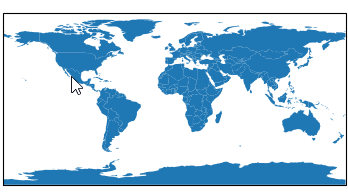# 💠 Vector Data

For vector data visualization, EOmaps utilizes the plotting capabilities of geopandas .

A `geopandas.GeoDataFrame` can be added to the map via `Maps.add_gdf()`. This is basically just a wrapper for the plotting capabilities of geopandas (e.g. GeoDataFrame.plot(…) ) supercharged with EOmaps features.

• If you provide a string or pathlib.Path object to `Maps.add_gdf()`, the contents of the file will be read into a `GeoDataFrame` via geopandas.read_file().

• Many file-types such as shapefile, GeoPackage, geojson … are supported!

 `Maps.add_gdf` Plot a geopandas.GeoDataFrame on the map.
```from eomaps import Maps
# import geopandas as gpd
# gdf = gpd.GeoDataFrame(geometries=[...], crs=...)<>

m = Maps()
# load the "ocean" data from NaturalEarth as a GeoDataFrame
# add the GeoDataFrame to the map
```

It is possible to make the shapes of a `GeoDataFrame` pickable (e.g. usable with `m.cb.pick` callbacks) by providing a `picker_name` (and specifying a `pick_method`).

• use `pick_method="contains"` if your `GeoDataFrame` consists of polygon-geometries (the default)

• pick a geometry if geometry.contains(mouse-click-position) == True

• use `pick_method="centroids"` if your `GeoDataFrame` consists of point-geometries

• pick the geometry with the closest centroid

Once the `picker_name` is specified, pick-callbacks can be attached via:

• `m.cb.pick[<PICKER NAME>].attach.< CALLBACK >()`

For example, to highlight the clicked country, you could use:
 ```from eomaps import Maps m = Maps() # get the GeoDataFrame for a given NaturalEarth feature gdf = m.add_feature.cultural.admin_0_countries.get_gdf(scale=110) # pick the shapes of the GeoDataFrame based on a "contains" query m.add_gdf(gdf, picker_name="countries", pick_method="contains") # temporarily highlight the picked geometry m.cb.pick["countries"].attach.highlight_geometry(fc="r", ec="g", lw=2) ```